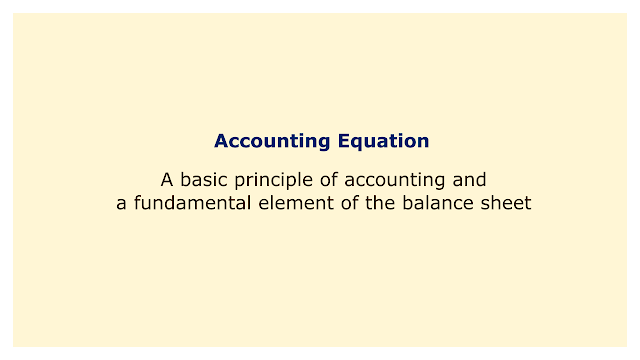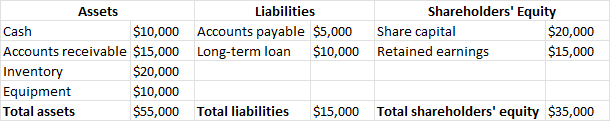# Accounting EquationImage: Moneybestpal.com

### The accounting equation is a cornerstone of balance sheet construction and a fundamental accounting principle. A balance sheet is a financial statement that lists a company's assets, liabilities, and shareholder's equity at a certain period.

The accounting equation ensures that these three elements are constantly balanced and demonstrates the relationship between them. The equation is as follows:

Assets = Liabilities + Shareholders' Equity

A company's assets are its valuable resources, such as cash, inventory, equipment, real estate, etc. Both current and non-current assets can be categorized as assets. Accounts receivable and marketable securities are examples of current assets that can be quickly converted into cash within a year. Non-current assets are those that, like land and buildings, are anticipated to be profitable for more than a year.

Liabilities are the debts or responsibilities a firm owes to other parties, including customers, lenders, lenders, employees, etc. Current obligations and non-current liabilities are two different categories of liabilities. Accounts payable and short-term loans are examples of current liabilities, which are obligations that have a one-year maturity date. Long-term loans and bonds are examples of non-current obligations because they are due more than a year from now.

Shareholders' equity is the amount of money that belongs to the owners or shareholders of the company. It indicates the company's remaining value after subtracting its obligations from its assets. Liabilities and assets can be subtracted to determine shareholders' equity, or share capital and retained earnings can be added to determine shareholders' equity. The sum of money that a firm has raised through the sale of shares to investors is known as share capital. Retained earnings are the accumulated profits that the business has kept for itself rather than giving dividends to shareholders.

The balance sheet is constantly balanced thanks in part to the accounting equation. In other words, for the equation to hold true, every transaction that changes one part of the equation must also change another part of the equation. For instance, if a business borrows money from a bank, its cash assets will grow by the same amount as its debt obligations (loan). When a business sells products to a consumer on credit, its assets (accounts receivable) will rise in proportion to its sales, boosting shareholders' equity (retained earnings).

To illustrate how the accounting equation works, let's look at an example of a simple balance sheet for a hypothetical company called ABC Inc.Image: Moneybestpal.com

As you can see, the accounting equation holds true for ABC Inc.:

\$55,000 = \$15,000 + \$35,000

The accounting equation is a helpful tool for comprehending and evaluating a company's financial status. It demonstrates how debt or equity is used to finance the company's assets. It also demonstrates how the components of the company's balance sheet are impacted by transactions. You may understand how accounting functions and how to read financial accounts by using the accounting equation.
Tags